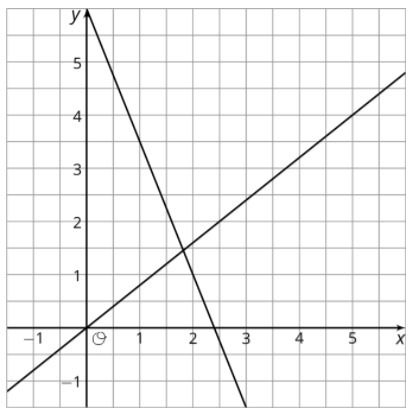PSABM5YC - Practice---Acc7.5 Lesson 16 Writing Systems of Equations (8.EE.C.8, 8.EE.C.8.b, 8.EE.C.8.c)
Part A)

Kiran and his cousin work during the summer for a landscaping company. Kiran's cousin has been working for the company longer, so his pay is 30% more than Kiran's. Last week his cousin worked 27 hours, and Kiran worked 23 hours. Together, they earned $493.85. What is Kiran's hourly pay? Do not include units ($) in your answer.

Type your answer below as a number (example: 5, 3.1, 4 1/2, or 3/2):
Part B)

Part A)

Decide which story can be represented by the system of equations and 100.

1. Diego’s teacher writes a test worth 100 points. There are 6 more multiple choice questions than short answer questions.
2. Lin and her younger cousin measure their heights. They notice that Lin is 6 inches taller, and their heights add up to exactly 100 inches.

Select one:
Part B)

Part A)

Clare and Noah play a game in which they earn the same number of points for each goal and lose the same number of points for each penalty. Clare makes 6 goals and 3 penalties, ending the game with 6 points. Noah earns 8 goals and 9 penalties and ends the game with -22 points.

Write a system of equations that describes Clare and Noah's outcomes. Use x to represent the number of points for a goal and y to represent the number of points for a penalty.

Part B)

Solve the system.

(x,y)

with no spaces.

Part C)

Solve:(x,y)

with no spaces.

Part A)

Estimate the coordinates of the point where the two lines meet.(x,y)

with no spaces.

Part B)

Choose two equations that make up the system represented by the graph.

1.2.3.4.5.Select all that apply:
Part C)

Solve the system of equations and confirm the accuracy of your estimate.

What is the x-value of the solution?